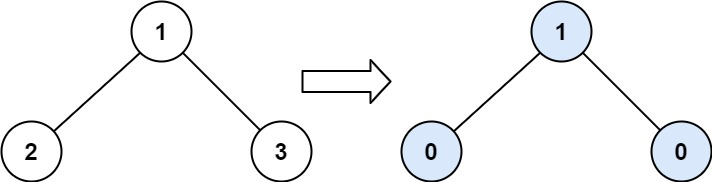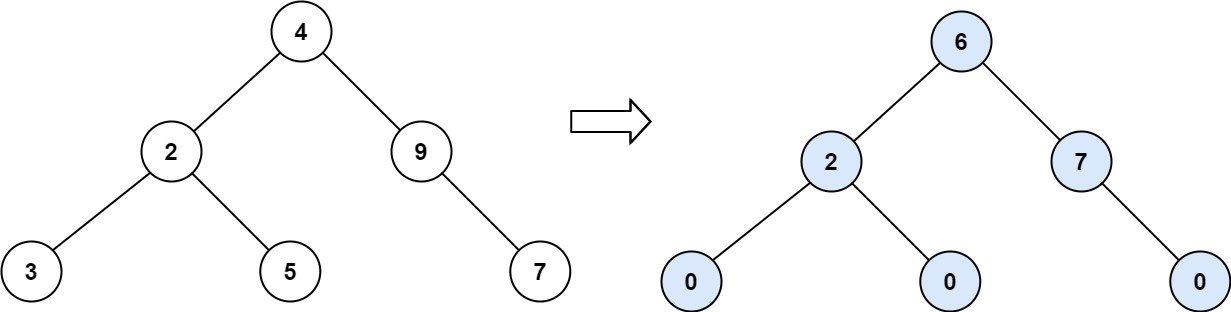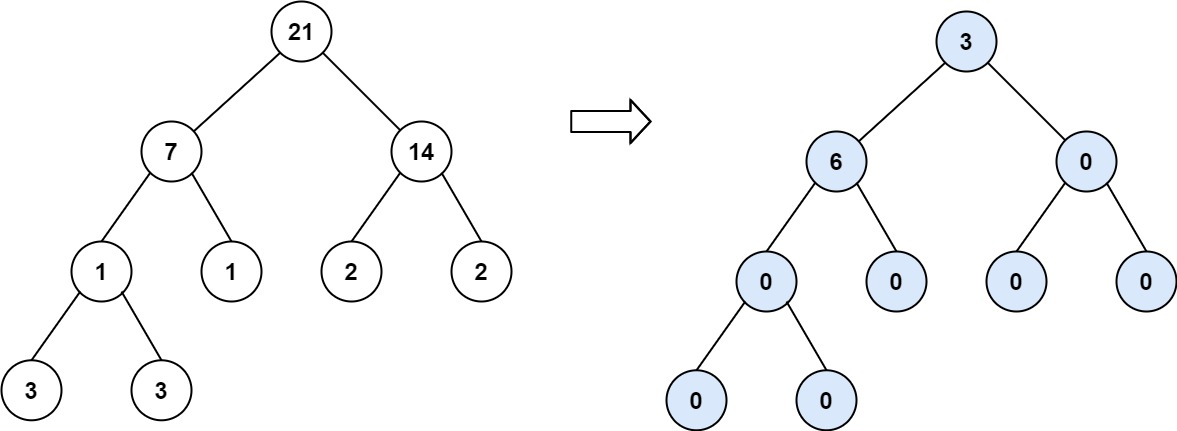## Algorithm

Problem Name: 563. Binary Tree Tilt

Given the `root` of a binary tree, return the sum of every tree node's tilt.

The tilt of a tree node is the absolute difference between the sum of all left subtree node values and all right subtree node values. If a node does not have a left child, then the sum of the left subtree node values is treated as `0`. The rule is similar if the node does not have a right child.

Example 1:```Input: root = [1,2,3]
Output: 1
Explanation:
Tilt of node 2 : |0-0| = 0 (no children)
Tilt of node 3 : |0-0| = 0 (no children)
Tilt of node 1 : |2-3| = 1 (left subtree is just left child, so sum is 2; right subtree is just right child, so sum is 3)
Sum of every tilt : 0 + 0 + 1 = 1
```

Example 2:```Input: root = [4,2,9,3,5,null,7]
Output: 15
Explanation:
Tilt of node 3 : |0-0| = 0 (no children)
Tilt of node 5 : |0-0| = 0 (no children)
Tilt of node 7 : |0-0| = 0 (no children)
Tilt of node 2 : |3-5| = 2 (left subtree is just left child, so sum is 3; right subtree is just right child, so sum is 5)
Tilt of node 9 : |0-7| = 7 (no left child, so sum is 0; right subtree is just right child, so sum is 7)
Tilt of node 4 : |(3+5+2)-(9+7)| = |10-16| = 6 (left subtree values are 3, 5, and 2, which sums to 10; right subtree values are 9 and 7, which sums to 16)
Sum of every tilt : 0 + 0 + 0 + 2 + 7 + 6 = 15
```

Example 3:```Input: root = [21,7,14,1,1,2,2,3,3]
Output: 9
```

Constraints:

• The number of nodes in the tree is in the range `[0, 104]`.

## Code Examples

### #1 Code Example with Java Programming

```Code - Java Programming```

``````
class Solution {
public int findTilt(TreeNode root) {
int[] total = {0};
helper(root, total);
}

private int helper(TreeNode root, int[] total) {
if (root == null) {
return 0;
}
int left = helper(root.left, total);
int right = helper(root.right, total);
total += Math.abs(left - right);
return left + right + root.val;
}
}
``````
Copy The Code &

Input

cmd
root = [1,2,3]

Output

cmd
1

### #2 Code Example with Javascript Programming

```Code - Javascript Programming```

``````
const findTilt = function(root) {
const tilt = { val: 0 }
dfs(root, tilt)
function dfs(root, tilt) {
if (!root) return 0
let left = dfs(root.left, tilt)
let right = dfs(root.right, tilt)
tilt.val += Math.abs(left - right)
return root.val + left + right
}
return tilt.val
}
``````
Copy The Code &

Input

cmd
root = [1,2,3]

Output

cmd
1

### #3 Code Example with Python Programming

```Code - Python Programming```

``````
class Solution:
def findTilt(self, root):
"""
:type root: TreeNode
:rtype: int
"""
def traverse(node):
if not node: return 0
left = traverse(node.left)
right = traverse(node.right)
res.append(abs(right -left))
return node.val + left + right
res = []
traverse(root)
return sum(res)
``````
Copy The Code &

Input

cmd
root = [4,2,9,3,5,null,7]

Output

cmd
15

### #4 Code Example with C# Programming

```Code - C# Programming```

``````
using System;

namespace LeetCode
{
public class _0563_BinaryTreeTilt
{
public int FindTilt(TreeNode root)
{
(_, int tilt) = ComputeSum(root);
return tilt;
}

private (int sum, int tilt) ComputeSum(TreeNode node)
{
if (node == null) return (0, 0);

(var leftSum, var leftTilt) = ComputeSum(node.left);
(var rightSum, var rightTilt) = ComputeSum(node.right);

var tile = Math.Abs(leftSum - rightSum);

return (leftSum + rightSum + node.val, tile + leftTilt + rightTilt);
}
}
}
``````
Copy The Code &

Input

cmd
root = [4,2,9,3,5,null,7]

Output

cmd
15